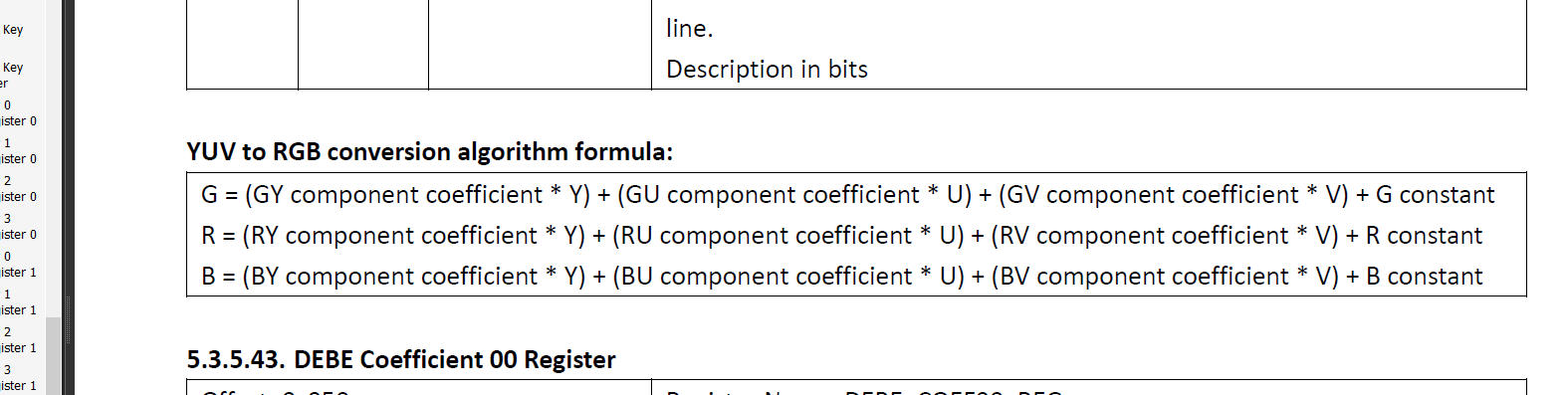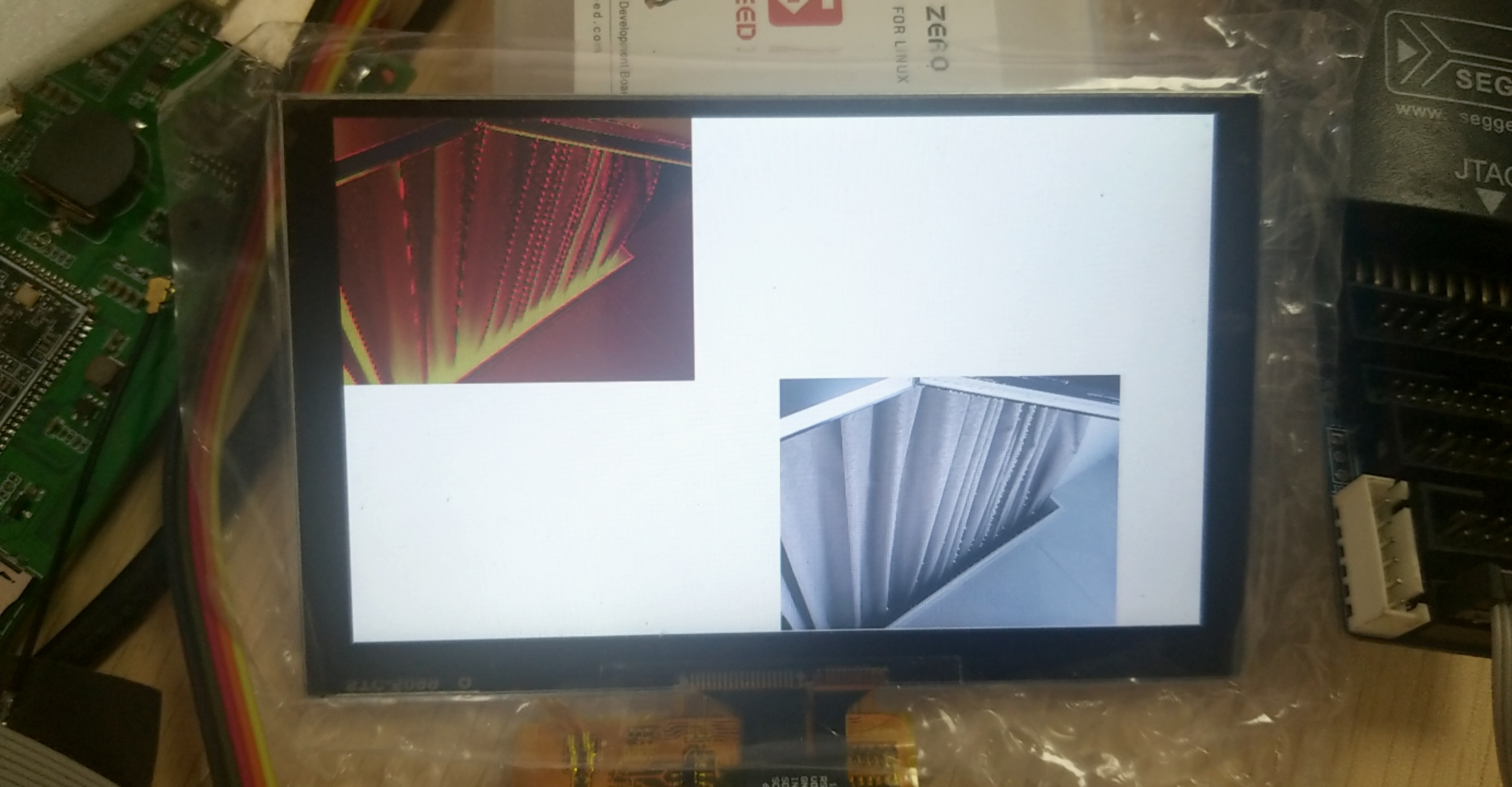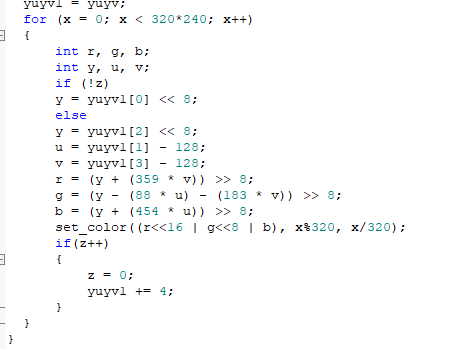# WhyCan Forum(哇酷开发者社区)

## #1 2020-10-27 17:03:57

vip888888

### F1c200s display engine 通过layer将YUV转RGB系数配置问题vip888888

vip888888 说：

## #5 2020-10-29 14:18:21

vip888888

### Re: F1c200s display engine 通过layer将YUV转RGB系数配置问题

r = (y + (359 * v)) >> 8;  = y/256 + (359*(V-128))/256 = y + (359*V - 45952)/256 = y+ 1.40234375*v - 179.5
g = (y - (88 * u) - (183 * v)) >> 8;  =y/256 -(88*(u-128))/256 - (183*(v-128))/256   = y -(88*u-11264)/256 - (183*v-23424)/256=y -0.34375u+44 -0.71484375*v + 91.5 = y-0.34375u-0.71484375*v + 135.5
b = (y + (454 * u)) >> 8;  = y/256 + (454*(u-128))/256 = y + (454u-58112)/256 = y + 1.7734375u - 227

r =y+ 1.40234375*v - 179.5
g =y-0.34375u-0.71484375*v + 135.5
b =y + 1.7734375u - 227f1c100_

vip888888

f1c100_ 说：

f1c100_

vip888888 说：
f1c100_ 说：

sprintf